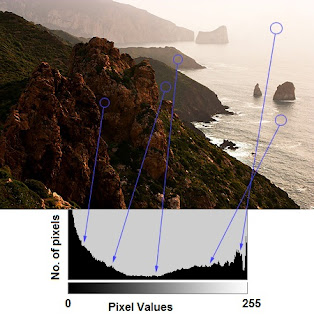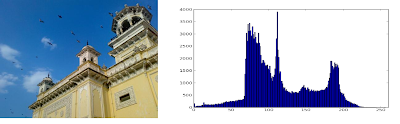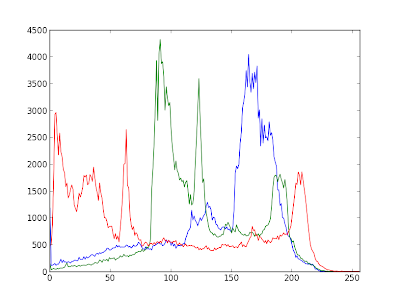# Histograms - 1 : Find, Plot, Analyze !!!

opencvpython.blogspot.com

## Histograms - 1 : Find, Plot, Analyze !!!

Hi,

This time, we will go through various functions in OpenCV related to histograms.

So what is histogram ? You can consider histogram as a graph or plot, which gives you an overall idea about the intensity distribution of an image. It is a plot with pixel values (ranging from 0 to 255) in X-axis and corresponding number of pixels in the image on Y-axis.

It is just another way of understanding the image. By looking at the histogram of an image, you get intuition about contrast, brightness, intensity distribution etc of that image. Almost all image processing tools today, provides features on histogram. Below is an image from "Cambridge in Color" website, and I recommend you to visit the site for more details.Image Histogram

You can see the image and its histogram. (Remember, this histogram is drawn for grayscale image, not color image). Left region of histogram shows the amount of darker pixels in image and right region shows the amount of brighter pixels. From the histogram, you can see dark region is more than brighter region, and amount of midtones (pixel values in mid-range, say around 127) are very less.

(For more basic details on histograms, visit : http://www.cambridgeincolour.com/tutorials/histograms1.htm)

FIND HISTOGRAM

Now we have an idea on what is histogram, we can look into how to find this. OpenCV comes with an in-built function for this, cv2.calcHist(). Before using that function, we need to understand some terminologies related with histograms.

BINS :
The above histogram shows the number of pixels for every pixel value, ie from 0 to 255. ie you need 256 values to show the above histogram. But consider, what if you need not find the number of pixels for all pixel values separately, but number of pixels in a interval of pixel values? say for example, you need to find the number of pixels lying between 0 to 15, then 16 to 31, ..., 240 to 255. You will need only 16 values to represent the histogram. And that is what is shown in example given in OpenCV Tutorials on histograms.

So what you do is simply split the whole histogram to 16 sub-parts and value of each sub-part is the sum of all pixel count in it. This each sub-part is called "BIN". In first case, number of bins where 256 (one for each pixel) while in second case, it is only 16. BINS is represented by the term "histSize" in OpenCV docs.

DIMS : It is the number of parameters for which we collect the data. In our case, we collect data regarding only one thing, intensity value. So here it is 1.

RANGE : It is the range of intensity values you want to measure. Normally, it is [0,256], ie all intensity values.

So now we use cv2.calcHist() function to find the histogram. Let's familiarize with the function and its parameters :
cv2.calcHist(images, channels, mask, histSize, ranges[, hist[, accumulate]])

1 - images : it is the source image of type uint8 or float32. it should be given in square brackets, ie, "[img]".
2 - channels : it is also given in square brackets. It the index of channel for which we calculate histogram. For example, if input is grayscale image, its value is . For color image, you can pass , or  to calculate histogram of blue,green or red channel respectively.
3 - mask : mask image. To find histogram of full image, it is given as "None". But if you want to find histogram of particular region of image, you have to create a mask image for that and give it as mask. (I will show an example later.)
4 - histSize : this represents our BIN count. Need to be given in square brackets. For full scale, we pass .
5 - ranges : this is our RANGE. Normally, it is [0,256].

So let's start with a sample image. Simply load an image in grayscale mode and find its full histogram.

`img = cv2.imread('home.jpg',0)hist = cv2.calcHist([img],,None,,[0,256])`

hist is a 256x1 array, each value corresponds to number of pixels in that image with its corresponding pixel value. Now we should plot it, but how ?

PLOTTING HISTOGRAM

There are two ways, 1) Short Way : use Matplotlib & 2) Long Way : use OpenCV functions

1 - Using Matplotlib:

Matplotlib comes with a histogram plotting function : matplotlib.pyplot.hist()

It directly finds the histogram and plot it. You need not use calcHist() function to find the histogram. See the code below:

`import cv2import numpy as npfrom matplotlib import pyplot as pltimg = cv2.imread('home.jpg',0)plt.hist(img.ravel(),256,[0,256]); plt.show()`

You will get a plot as below :Image Histogram

NOTE : Actually to find histogram, Numpy also provides you a function, np.histogram(). So instead of calcHist() function, you can try below line :

hist,bins = np.histogram(img,256,[0,256])

hist is same as we calculated before. But bins will have 257 elements, because numpy calculate bins as 0-0.99,1-1.99,2-2.99 etc. So final range would be 255-255.99. To represent that, they also add 256 at end of bins. But we don't need that 256. Upto 255 is sufficient.

Or you can use normal plot of matplotlib, which would be good for BGR plot. For that, you need to find the histogram data first. Try below code:

`import cv2import numpy as npfrom matplotlib import pyplot as pltimg = cv2.imread('home.jpg')color = ('b','g','r')for i,col in enumerate(color):    histr = cv2.calcHist([img],[i],None,,[0,256])    plt.plot(histr,color = col)    plt.xlim([0,256])plt.show()`

You will get a image as below :Histogram showing different channels

You can deduct from the above graph that, blue has some high value areas(obviously it should be the due to sky)

2 - Using OpenCV functions :

Well, here you adjust the values of histograms along with its bin values to look like x,y coordinates so that you can draw it using cv2.line() or cv2.polyline() function to generate same image as above. This is already available with OpenCV-Python2 official samples. You can check that : https://github.com/Itseez/opencv/blob/master/samples/python2/hist.py . I had already mentioned it in one of my very early articles : Drawing Histogram in OpenCV-Python

Now we used calcHist to find the histogram of full image. What if you want to find some regions of an image? Just create a mask image with white color on the region you want to find histogram and black otherwise. I have demonstrated it while answering a SOF question. So I would like you to read that answer (http://stackoverflow.com/a/11163952/1134940). Just for a demo, I provide the same images here :Application of Mask
Due to resizing, histogram plot clarity is reduced. But I hope you can write your own code and analyze it.

SUMMARY
In short, we have seen what is image histogram, how to find and interpret histograms, how to plot histograms etc. It is sufficient for today. We will look into other histogram functions in coming articles.

Hope you enjoyed it !!! Feel free to share !!!

Abid Rahman K.

# 3 Comments on OpenCV-Python: Histograms - 1 : Find, Plot, Analyze !!!

•on March 07, 2013 | 03:06 Thorsaid :
"Thank you!"
•on March 11, 2013 | 21:46 MATHERsaid :
"Hi, can any one give me or tell me how to face detection in python 2.7 with opencv 2.4.3
and Window 7
"
•on March 12, 2013 | 00:18 abidksaid :
"ready code is available in opencv official samples. check them."

## Report "Histograms - 1 : Find, Plot, Analyze !!!"

Are you sure you want to report this post for ?

Cancel
×